# CBSE Class 10 Maths Chapter 6 Triangles Objective Questions

CBSE Class 10 Maths Chapter 6 Triangles Objective Questions explain concepts such as Introduction to Triangles, Similarity of Triangles, Areas of Similar Triangles, Pythagoras Theorem, and so on. An important part of Unit 3 – Geometry, Chapter 6 – Triangles have nine theorems in total. Having a clear understanding of the concepts, theorems, and problem-solving methods in this chapter is mandatory to score well in the board examination of Class 10 Maths. Taking this into consideration, along with the fact that the changed exam pattern will include more MCQs, we have compiled the CBSE Class 10 Maths Chapter 6 – Triangles Objective Questions for the students to solve and practise. The more they practise, the more they master the concepts.

### List of Sub-Topics Covered in Chapter 6

6.1 Areas of Similar Triangles (4 MCQs from the Topic)

6.2 Basic Proportionality Theorem (4 MCQs from the Topic)

6.3 Criteria for Similarity of Triangles (4 MCQs from the Topic)

6.4 Pythagoras Theorem (4 MCQs from the Topic)

6.5 Similar Triangles (4 MCQs from the Topic)

The CBSE Class 10 Maths Objective Questions covering the above topics will help the students to prepare well for the board exams.

## Download CBSE Class 10 Maths Chapter 6 – Triangles Objective Questions Free PDF

### Areas of Similar Triangles

1. If △ ABC ~ △ DEF such that AB = 12 cm and DE = 14 cm, find the ratio of areas of △ ABC and △ DEF.
1. 49/9
2. 36/49
3. 49/16
4. 25/49

Solution: We know that the ratio of areas of two similar triangles is equal to the ratio of the squares.

For any two corresponding sides,

The area of △ ABC / area of △ DEF = (AB/DE) 2= (12/14) 2= 36/49

1. D and E are points on the sides AB and AC, respectively, of a △ABC such that DE || BC. Which of the following statement is true?
1. △ ADE ~ △ ABC
1. only (iii)
2. only (i)
3. only (i) and (ii)
4. all (i) , (ii) and (iii)

Solution: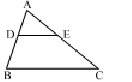In △ ADE and △ ABC, we have

[Since DE || BC ∠ ADE = ∠ B (Corresponding angles)], and ∠ A = △ A [Common]

1.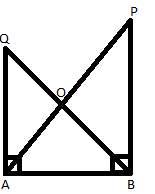In the figure, PB and QA are perpendicular to segment AB. If OA = 5 cm, PO = 7cm, and area (ΔQOA) = 150 cm2, find the area of ΔPOB.

1. 233 cm2
2. 294 cm2
3. 300 cm2
4. 420 cm2

Solution: Consider Δ~QOA and Δ POB

QA || PB,

Therefore, ∠ AQO = ∠ PBO [Alternate angles]

∠ QAO = ∠ BPO  [Alternate angles]

and

∠ QOA = ∠ BOP [Vertically opposite angles]

Δs QOA ~ BOP  [by AAA similarity]

Therefore, (OQ/ OB) = (OA/OP)

Now, area (POB)/ area (QOA) = (OP) 2/ (OA) 2= 72/ 52

Since area (QOA) = 150cm2

⇒area (POB) = 294cm2

1. Two isosceles triangles have equal angles, and their areas are in the ratio 16:25. The ratio of corresponding heights is:
1. 4:5
2. 5:4
3. 3:2
4. 5:7

Solution: For similar isosceles triangles,

Area (Δ1) / Area (Δ2) = (h1)2 / (h2)2

(h1 / h2) = 4/5

### Basic Proportionality Theorem

1. In △ABC, AB = 3 and AC = 4 cm, and AD is the bisector of ∠A. Then, BD:DC is:
1. 9:16
2. 4:3
3. 3:4
4. 16:9

Solution: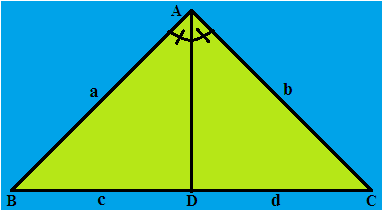The angle bisector theorem states that if a ray bisects an angle of a triangle, then it divides the opposite side into segments that are proportional to the other two sides (It may be similar or may not depending on the type of triangle it divides).

In △ABC, as per the statement AB/ AC= BD/DC, i.e., a/b= c/d

So, BD/DC= AB/AC= ¾

So, BD:DC = 3:4

1. ABCD is a parallelogram with diagonals AC if a line XY is drawn such that XY ∥ AB.

BX/XC=?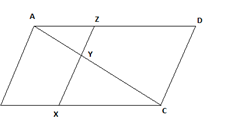1. (AY/AC)
2. DZ/AZ
3. AZ/ZD
4. AC/AY

Solution: In the Δ ABC,

AB ∥ XZ

AB ∥ XY

∴ BX/ XC= AY/YC…. (By BPT)….. (1)

In parallelogram ABCD,

AB ∥ CD

AB ∥ CD ∥ XZ

In the Δ ACD,

CD ∥ YZ

∴ AY/YC= AZ/ZD … (By BPT)…… (2)

From 1 & 2,

BX/XC= AY/YC= AZ/ZD

BX/XC= AZ/ZD

1.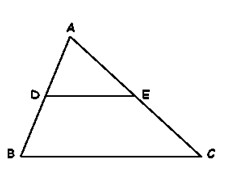In ABC, given that DE//BC, D is the midpoint of AB, and E is the midpoint of AC.

The ratio AE:EC is ____.

A. 1:3

B. 1:1

C. 2:1

D. 1:2

Solution: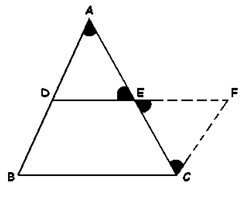DE is parallel to BC

∠DAE = ∠ECF {Alternate angles}

∠BAC = ∠DAE

⇒ AD/DB= AE/EC (Basic Proportionality Theorem)

Since D is the midpoint of AB,

⇒ AE/EC= 1 /1

∴ AE:EC = 1:1

1. In ΔABC, AC = 15 cm and DE || BC. If AB/AD=3, find EC.
1. 5 cm
2. 10 cm
3. 2.5 cm
4. 9 cm

Solution: Given: DE∥BC

From the basic proportionality theorem,

⇒2AE=EC⇒AC=AE+EC−−−−−−− (1)

On substituting the value of EC in (1), we get

15=3AE⇒5=AE⇒EC=10 cm

### Criteria for Similarity of Triangles

1. △ ABC is an acute-angled triangle. DE is drawn parallel to BC, as shown. Which of the following is always true?

i) △ ABC ∼ △ ADE

iii) DE= BC/2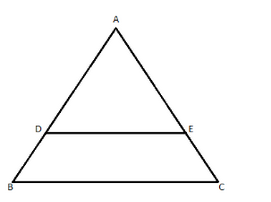1. Only (i)
2. (i) and (ii) only
3. (i), (ii) and (iii)
4. (ii) and (iii) only

Answer: (B) (i) and (ii) Only

DE = BC/2 only if D and E are the midpoints of AB and AC, respectively. So this may not always be true.

1. The triangles ABC and ADE are similar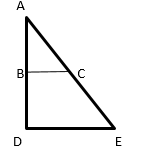Which of the following is true?

2. BC/BD=CE/DE
4. All of the Above

Solution: Since the given triangles are similar, the ratios of the corresponding sides are equal.

1. If in △ CAB and △ FED, AB/ EF=BC/FD=AC/ED, then:
1. △ ABC∼△ DEF
2. △ CAB∼△ DEF
3. △ ABC∼△ EFD
4. △ CAB∼△ EFD

Solution: If two triangles are similar, the corresponding sides are proportional.

Therefore, △ABC∼△EFD.

1. A tower of height 24m casts a shadow of 50 m, and at the same time, a girl of height 1.8 m casts a shadow. Find the length of the shadow of the girl.
1. 3.75 m
2. 3.5 m
3. 3.25 m
4. 3 m

Solution: In △ABC and △DEC

∠ABC=∠DEC=90°

∠C=∠C (common)

Therefore, △ABC∼△DEC       [by AA similarity]

So, DE/AB=EC/BC

EC=DE × (BC/AB)

EC= 1.8× (50/24) ⇒EC=3.75 m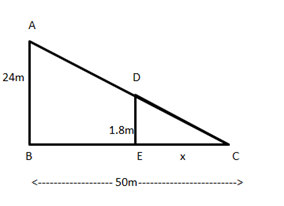### Pythagoras Theorem

1. In the adjoining figure, if BC = a, AC = b, AB = c and  ∠CAB = 120°, then the correct relation is: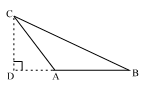1. a2 = b2 +  c2 – bc
2. a2 = b2 +  c2 + bc
3. a2 = b2 +  c2 – 2bc
4. a2 = b2 +  c+ 2bc

Answer: (B) a2 = b2 +  c2 + bc

Solution: In △CDB,

BC2 =  CD2 + BD2      [By Pythagoras Theorem]

BC2 =  CD2 + (DA+AB)

BC2 =  CD2 + DA2 + AB2 + (2×DA×AB)         (i)

CD2 + DA2 = AC2      (ii)  [By Pythagoras Theorem]

Putting the values from (ii) and (iii) in (i), we get

BC2 =  AC2 + AB2 + (AC×AB)

a2 = b2 +  c2 + bc

Alternatively,

Since  ∠A is an obtuse angle in  ΔABC,

BC2 =  AB2 +  AC2 + 2AB . AD

= AB2 +  AC2 + 2×AB× ½ ×AC

[∵ AD = AC cos  60∘ = 1/2AC]

= AB2 +  AC2 + AB × AC

a2 = b2 +  c2 + bc.

1. If the distance between the top of two trees 20 m and 28 m tall is 17 m, then the horizontal distance between the trees is:
1. 11 m
2. 31 m
3. 15 m
4. 9 m

Solution: Let AB and CD be two trees such that AB = 20 m, CD = 28 m & BD = 17 m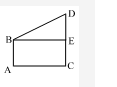Draw BE parallel to CD. Then, ED = 8 m.

By applying the Pythagoras theorem:

BE2+DE2=BD2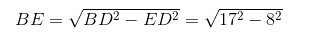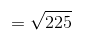=15m

∴ AC = BE = 15 m

1.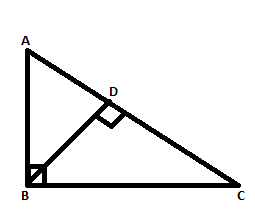In the figure, △ABC is a right-angled triangle with a right angle at B, and BD is perpendicular to AC. Then which of the following options will hold true?

Solution: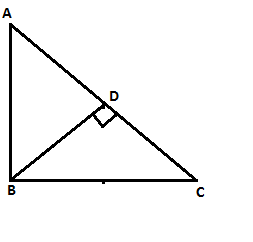∠A=∠A (common angle)

1. In a right △ABC, a perpendicular BD is drawn to the largest side from the opposite vertex. Which of the following does not give the ratio of the areas of △ABD and △ACB?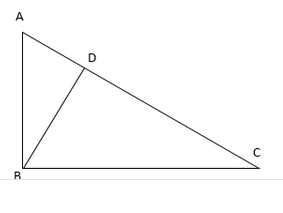1. (AB/AC)2
4. (BD/CB)2

Solution:

Consider  ΔABD and ΔACB: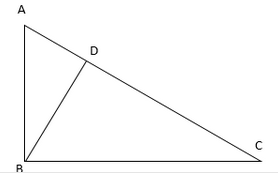∠BDA =  ∠ABC     [ 90°]

By AA similarity criterion, △ABD ~ △ACB

Hence,

### Similar Triangles

1. △ ABC is such that AB = 3 cm, BC = 2 cm, and CA = 2.5 cm. △ DEF is similar to △ABC. If EF = 4 cm, then the perimeter of △DEF is:
1. 7.5 cm
2. 15 cm
3. 30 cm
4. 22.5 cm

Solution: AB/DE= AC/DF=BC/EF=2/4=1/2

DE = 2×AB = 6 cm, DF = 2×AC = 5 cm

∴ The perimeter of △DEF = (DE + EF + DF) = 15 cm.

1. In △ ABC and △ DEF, ∠A = ∠E = 40∘ and AB/ED=AC/EF. Find ∠B if ∠F is 65°.
1. 85°
2. 75°
3. 35°
4. 65°

Solution: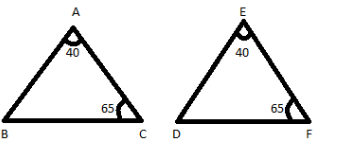AB/ED= AC/EF (Given)

∠A = ∠E = 40°

Since the ratio of adjacent sides and the included angles are equal,

△ABC is similar to △EDF by the SAS similarity criterion.

Now, ∠C = ∠F = 65°       [Corresponding angles of similar triangles are equal]

∴ ∠B = 180°− (∠A+∠C)

=180° – (40° + 65°) = 75°

1. The ratio of the corresponding sides of two similar triangles is 1:3. The ratio of their corresponding heights is:
1. 1:3
2. 3:1
3. 1:9
4. 9:1

Solution: Ratio of heights = Ratio of sides = 1:3.

1. If Δ ABC and Δ DEF are similar such that 2AB = DE and BC = 8 cm, then find EF.
1. 16 cm
2. 12 cm
3. 8 cm
4. 4 cm

Solution: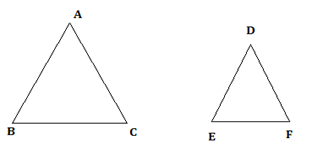2AB = DE

2BC = EF

⇒ 2×8 = EF

⇒ EF = 16 cm

## CBSE Class 10 Maths Chapter 6 Extra MCQs

1. A square and a rhombus are always _____.
(a) congruent
(b) similar but congruent
(c) similar
(d) neither similar nor congruent

2. What is the length of each side of a rhombus whose diagonals have lengths of 10 cm and 24 cm?
(a) 13 cm
(b) 26 cm
(c) 25 cm
(d) 34 cm

Triangle is the most interesting and exciting chapter of Unit 3 – Geometry, as it takes the students through the different aspects and concepts related to the geometrical figure triangle. A triangle is a plane figure with three sides and three angles. This chapter deals with several topics and sub-topics related to triangles.

Get more such CBSE Class 10 Maths and Science notes, at BYJU’S. Also, access Class 10 question papers, sample papers, and other study materials to prepare for the board exam in a more effective way.